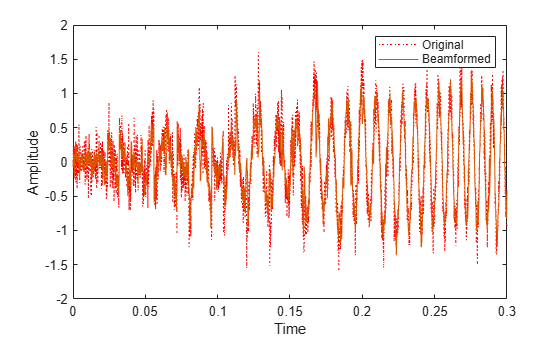# step

System object: phased.TimeDelayLCMVBeamformer
Package: phased

Perform time-delay LCMV beamforming

## Syntax

```Y = step(H,X) Y = step(H,X,XT) Y = step(H,X,ANG) [Y,W] = step(___) ```

## Description

Note

Starting in R2016b, instead of using the `step` method to perform the operation defined by the System object™, you can call the object with arguments, as if it were a function. For example, ```y = step(obj,x)``` and `y = obj(x)` perform equivalent operations.

`Y = step(H,X)` performs time-delay LCMV beamforming on the input, `X`, and returns the beamformed output in `Y`. `X` is an M-by-N matrix where N is the number of elements of the sensor array. M must be larger than the FIR filter length specified in the `FilterLength` property. `Y` is a column vector of length M.

The size of the first dimension of the input matrix can vary to simulate a changing signal length. A size change can occur, for example, in the case of a pulse waveform with variable pulse repetition frequency.

`Y = step(H,X,XT)` uses `XT` as the training samples to calculate the beamforming weights when you set the `TrainingInputPort` property to `true`. `XT` is an M-by-N matrix where N is the number of elements of the sensor array. M must be larger than the FIR filter length specified in the `FilterLength` property.

`Y = step(H,X,ANG)` uses `ANG` as the beamforming direction, when you set the `DirectionSource` property to `'Input port'`. `ANG` is a column vector of length 2 in the form of `[AzimuthAngle; ElevationAngle]` (in degrees). The azimuth angle must be between –180° and 180°, and the elevation angle must be between –90° and 90°.

You can combine optional input arguments when their enabling properties are set: `Y = step(H,X,XT,ANG)`

`[Y,W] = step(___)` returns additional output, `W`, as the beamforming weights when you set the `WeightsOutputPort` property to `true`. `W` is a column vector of length L, where L is the number of degrees of freedom of the beamformer. For a time-delay LCMV beamformer, the number of degrees of freedom is given by the product of the number of elements of the array and the filter length specified by the value of the `FilterLength` property.

Note

The object performs an initialization the first time the object is executed. This initialization locks nontunable properties and input specifications, such as dimensions, complexity, and data type of the input data. If you change a nontunable property or an input specification, the System object issues an error. To change nontunable properties or inputs, you must first call the `release` method to unlock the object.

All input and output arguments can be single or double precision.

## Examples

expand all

Apply a time delay LCMV beamformer to an 11-element acoustic ULA array. The elements are omnidirectional microphones. The incident angle of the signal is -50 degrees in azimuth and 30 degrees in elevation. The incident signal is an FM chirp with 500 Hz bandwidth. The propagation speed is a typical speed of sound in air, 340 m/s.

Simulate the signal and add noise.

```nElem = 11; microphone = phased.OmnidirectionalMicrophoneElement(... 'FrequencyRange',[20 20000]); array = phased.ULA('Element',microphone,'NumElements',nElem,'ElementSpacing',0.04); fs = 8000; t = 0:1/fs:0.3; x = chirp(t,0,1,500); c = 340; collector = phased.WidebandCollector('Sensor',array,... 'PropagationSpeed',c,'SampleRate',fs,... 'ModulatedInput',false); incidentAngle = [-50;30]; x = collector(x.',incidentAngle); noise = 0.2*randn(size(x)); rx = x + noise;```

Create and apply the time-delay LCMV beamformer. Specify a filterlength of 5.

```filterLength = 5; constraintMatrix = kron(eye(filterLength),ones(nElem,1)); desiredResponseVector = eye(filterLength,1); beamformer = phased.TimeDelayLCMVBeamformer('SensorArray',array,... 'PropagationSpeed',c,'SampleRate',fs,'FilterLength',filterLength,... 'Direction',incidentAngle,'Constraint',constraintMatrix,... 'DesiredResponse',desiredResponseVector); y = beamformer(rx);```

Compare the beamformer output to the input to the middle sensor.

```plot(t,rx(:,6),'r:',t,y) xlabel('Time') ylabel('Amplitude') legend('Original','Beamformed')```## Algorithms

The beamforming algorithm is the time-domain counterpart of the narrowband linear constraint minimum variance (LCMV) beamformer. The algorithm does the following:

1. Steers the array to the beamforming direction.

2. Applies an FIR filter to the output of each sensor to achieve the specified constraints. The filter is specific to each sensor.